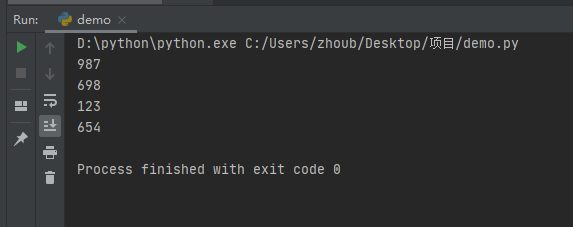• 写文章
• 提问

# 如何使用python3中的print输出整数？1. print中用 %d 输出

```>>> print("%d + %d = %d" % (1,2,3))
1 + 2 = 3
>>>```

2. str()函数将数字转换

```x = [23.5, 45.6, 987, 698, 123, 654]
for i in x:
i = str(i)
if i.count('.') == 0:
print(I)``````f = 1.6
print("1.6直接整型输出是      %d"%f,end="\n")
print("1.6利用浮点数的精度范围输出%.0f"%f)```

1.6直接整型输出是 1

1.6利用浮点数的精度范围输出2